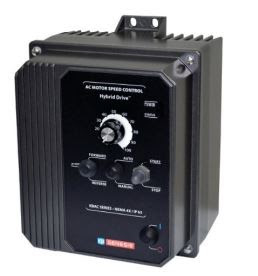VFD | Source: KB Electronics

The most important consideration when choosing a Variable Frequency Drive (VFD) is to know the type of load that is being applied to the motor because it will determine the size and cost of the VFD. The VFD must have sufficient current capability so that the motor can produce the required torque for the load. KB Electronics offers VFDs for AC induction, Permanent Magnet Synchronous Motors (PMSM), and Brushless DC (BLDC) motors that can operate efficiently for various types of loads.

Knowing the load profile (speed range, torque, and power) is essential when selecting a suitable motor and VFD for the application. Generally, loads can be grouped into five categories:

The size of the load, the required speed, and acceleration/deceleration will define the torque and horsepower that is required.

The basic equation that relates torque, speed, and horsepower is:

Horsepower = Torque x Speed

Related Article: Variable Frequency Drive and the Affinity Law

With constant torque loads, torque is not a function of speed. As the speed changes, torque remains constant and the horsepower changes linearly with speed.

Constant torque loads cause motors to draw relatively high current at low speeds when compared to variable torque applications. For this kind of use, the VFD must be able to provide a high starting torque (1.5 times or more the nominal rate) to overcome static friction and inertia of the load.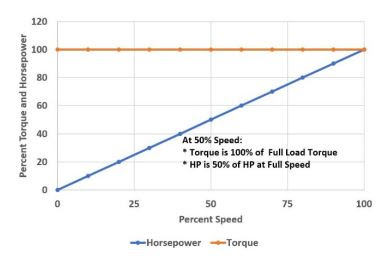Torque – Speed Profile of Constant Torque LoadsSource: KB Electronics

The figure above shows the Torque-Speed and Horsepower-Speed plot for Constant Torque loads. The torque remains constant up to the base/full speed.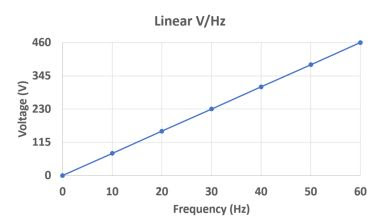Volts per Hertz plot

In order to keep a constant torque, which is directly related to current, the voltage going from the VFD to the motor is increased as the speed increases. On a 460 Volt motor, 460 Volts is only applied to the motor when the speed reaches 60 Hz. Constant torque is achieved because the VFD is increasing the voltage to the motor as it increases the speed.

This is displayed by the Volts/Hz chart shown in Figure 2. In this plot, when the VFD is set to 30 Hz, it applies 230 Volts to the motor and when the VFD is set to 60 Hz, 460 Volts is applied to the motor. This relationship keeps the current and torque to the motor relatively constant.

Typical applications are:

• Traction Drives
• Compressors, Conveyors
• Mixers
• Hoists.

Variable torque loads require much lower torque at low speeds and the torque increases rapidly at high speeds. As the motor spins faster, the load on the motor increases. These types of loads are approximately represented by assuming that the load torque required is proportional to the square of the speed. For example, if speed increases by a factor of 3, torque increases by a factor of 9.

• Torque is directy proportional to the square of speed.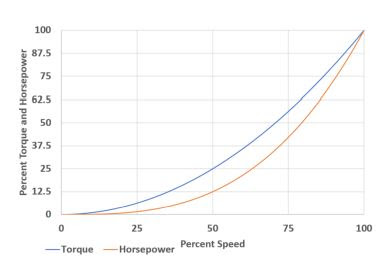Torque – Speed Profile of Variable Torque LoadsSource: KB Electronics

Consider an example of a HVLS fan that uses a 2 HP motor to deliver a torque of 100 Nm at base speed of 100 RPM. When the speed of the fan is reduced by half (or 50 RPM), the torque required to drive the fan blades is reduced to one-quarter (or 25 Nm). The horsepower is reduced to the speed cubed, which is 1/8, or 0.25 HP of that required to drive the load at 100 RPM.

For some machines, the torque required decreases as the speed increases. This particularly applies to constant horsepower operation when the motor provides a torque that is inversely proportional to the speed of the motor. With these loads, the power is constant so there will rarely be energy savings when the speed is reduced. For constant horsepower loads, torque is inversely proportional to speed. Above base speed, the voltage supplied to the motor cannot be increased as it reaches its maximum limit. As the drive continues to increase the speed, and with the voltage staying the same, the current to the motor starts to drop off, which means that the torque drops as well. Such applications include center-driven constant torque winders and log peeling lathes.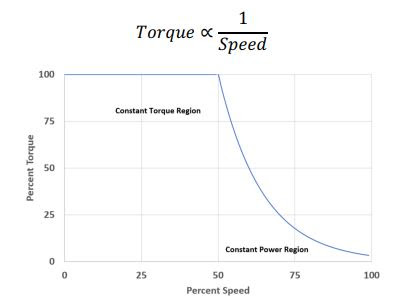Torque – Speed Profile of Constant Power LoadsSource: KB Electronics

The figure above shows the Torque-Speed profile of Constant Power Loads. Above base speed, the motor enters the constant power region where the torque decreases inversely with increase in speed. Consider a winder. A product is being wound onto a drum at a constant linear speed v, and constant tension, T. As the number of windings increase, the radius, r of the product being wound also increases, and torque also increases to maintain the constant tension. Also, to maintain the constant linear speed v, the rotational speed ω, decreases as v = ω x r.

Impact loads demand torque intermittently and do not depend on motor speed. Consider a punch press, which uses a large flywheel to deliver the mechanical energy needed for the load. Press applications require that the motor and VFD combination produce enough accelerating torque to return the flywheel to the required speed prior to the beginning of the next work stroke.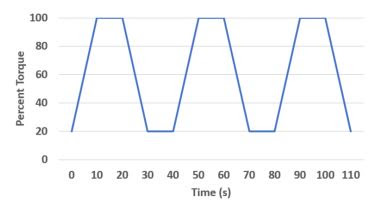Impact Loads

The figure above shows the Torque vs. Time Curve for impact loads.

Loads that have high inertia demand more torque at the start. Therefore, breakaway torque is the initial torque that is required to move a load. It defines the starting torque of the motor and the output current rating of the VFD. If the breakaway torque is not considered, the VFD might trip due to current limit and the motor cannot start the load. Current limit is set based on the breakaway load. In general, the breakaway torque is expressed as a percentage of full-load torque.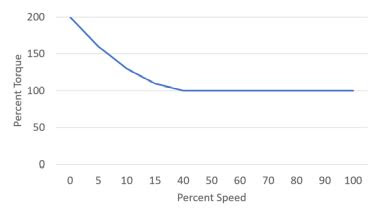Torque – Speed Profile of Breakaway LoadsSource: KB Electronics

The figure above shows the Torque – Speed profile of Breakaway type loads. The starting torque is around 200% of full load torque. Consider an example of a mixer that contains a thick and viscous fluid substance. When the VFD starts to turn the motor, the viscosity of the substance demands more torque from the motor to spin the fluid. The VFD increases the current output up to 200% for a few seconds depending upon the viscosity to get the mixer started. As the load starts spinning, the torque and current reduces to the rated values. If the load does not move, the VFD trips for protection of the VFD, motor, and mixer.

Source:

• How to Select a Variable Frequency Drive Based on Load Characteristics
• Author: Vishnuvarthanaraj (Vishnu) Balaraj, Software/Hardware Engineer